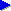HomeIntroductionComplex numbersNewton's methodNewton's method with complex numbersFractalsOptimisationsPerformanceSearch this site: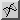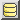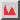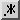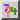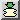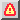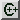Got a question about Java? Java discussion forum

# The cost of Java object orientation in mathematical calculations: an example with Newton's method fractals

In various program designs, an important question arises: to what extent should data be encapsulated into separate objects, and what affect this encapsulation will have on performance? The question becomes particularly crucial when the most obvious encapsulation from a program design and maintenance point of view would lead to a large number of objects being created and to rapid object throughput (the creation of a relatively large number of objects in a given space of time).

In this article, I examine the case of calculation with complex numbers. If you are not familiar with the concept of complex numbers, essentially they involve a type of calculation where:

• the most intuitive and maintainable implementation would lead to rapid object throughput as numerous objects are created in intermediate steps of a calculation;
• a less intuitive and less maintainable implementation is generally possible that "optimises away" the use of objects in intermediate calculations.

So the question we essentially address is: how much worse in terms of performance is the maintainable, object-oriented solution compared to the "hand-cranked" optimisation that avoids object creation?

Our test task will consist of generating a fractal image based on Newton's method, as in Figure 1 below: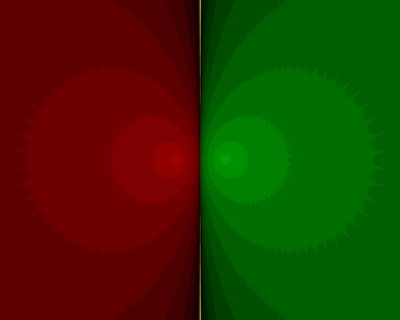This is essentially a computationally expensive task that relies on complex numbers and, in the case of the formula that we choose to generate the image, it is relatively straightforward to create an optimised version that avoids object encapsulation. We find that:

• encapsulating complex numbers as immutable objects is roughly 2.75 times slower than an optimised version of the algorithm that avoids object creation;
• encapsulating complex numbers as mutable objects, and thus avoiding some of the object creation involved in intermediate calculations, is roughly twice as slow as the optimised version.

On the next pages, we look at the background to the problem, with an overview of complex numbers and Newton's method. If you're familiar with these two concepts, you may want to skip forward to the section on fractals with Newton's method, or even skip straight forward to the section discussing optimisation and performance measurements of the object oriented approach to complex number calculation.

Unless otherwise stated, the Java programming articles and tutorials on this site are written by Neil Coffey. Suggestions are always welcome if you wish to suggest topics for Java tutorials or programming articles, or if you simply have a programming question that you would like to see answered on this site. Most topics will be considered. But in particular, the site aims to provide tutorials and information on topics that aren't well covered elsewhere, or on Java performance information that is poorly described or understood. Suggestions may be made via the Javamex blog (see the site's front page for details).